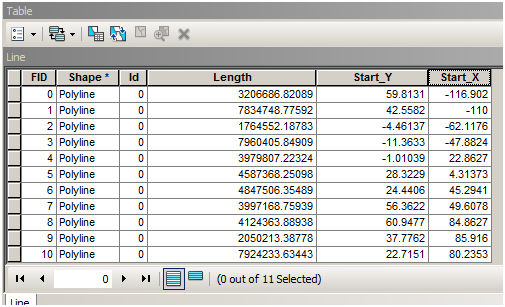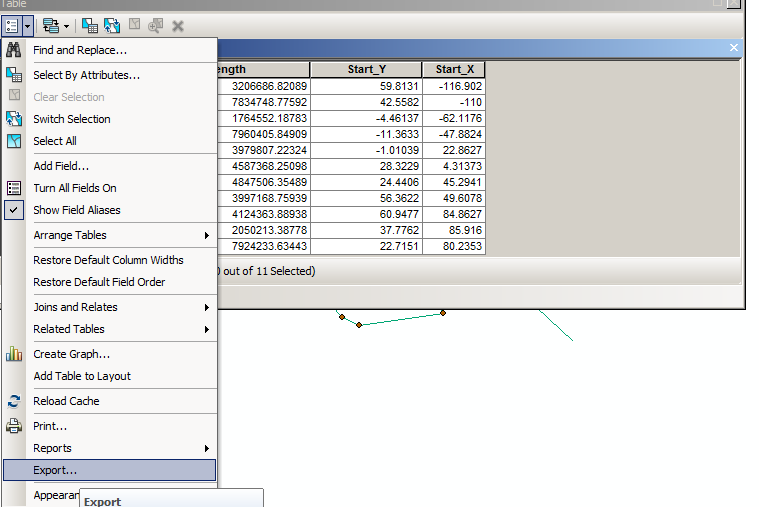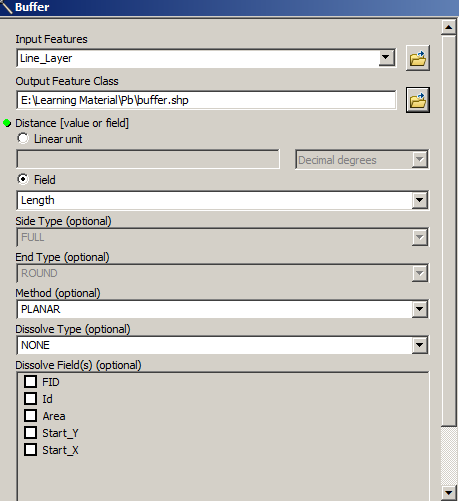# Polyline to Circle

4015
3
02-20-2015 12:50 AMNew Contributor

Hi,

I have a layer of 120 polylines created from XY coordinates of start and end points.  I now would like to create a layer of circles (polylines not polygons) with one end point of each polyline as the center and the other end point of the polyline as the outer radius.

How do I do this?

Thanks!

Tags (3)
1 Solution

Accepted SolutionsbyMVP Esteemed Contributor

Hi,

You could follow the following workflow.

1) Add 3 fields to the polyline layer, viz., Length (in meters), Start_X (X Coordinate/Longitude of Starting point), and Start_Y (Y Coordinate/Latitude of Starting point) using Calculate Geometry in the attribute table.2) Export the attribute table to a table file (e.g. *.dbf)3) In ArcCatalog, create XY point feature class from the above table file. Define a GCS spatial reference.4) Reproject the shapefile to a projected coordinate system (e.g. UTM).

5) Use Buffer (Analysis) tool to build the Circles, as desired.Note: We are reprojecting the points to PCS, so that the buffer takes up linear units (meters) and not angular units (Degree Decimal).

I hope it solves your issue.

Thanks,

Jay

Think Location
3 RepliesbyMVP Esteemed Contributor

Hi,

You could follow the following workflow.

1) Add 3 fields to the polyline layer, viz., Length (in meters), Start_X (X Coordinate/Longitude of Starting point), and Start_Y (Y Coordinate/Latitude of Starting point) using Calculate Geometry in the attribute table.2) Export the attribute table to a table file (e.g. *.dbf)3) In ArcCatalog, create XY point feature class from the above table file. Define a GCS spatial reference.4) Reproject the shapefile to a projected coordinate system (e.g. UTM).

5) Use Buffer (Analysis) tool to build the Circles, as desired.Note: We are reprojecting the points to PCS, so that the buffer takes up linear units (meters) and not angular units (Degree Decimal).

I hope it solves your issue.

Thanks,

Jay

Think LocationbyMVP Esteemed Contributor

Forgot to add my output snapshot!!!Think LocationNew Contributor